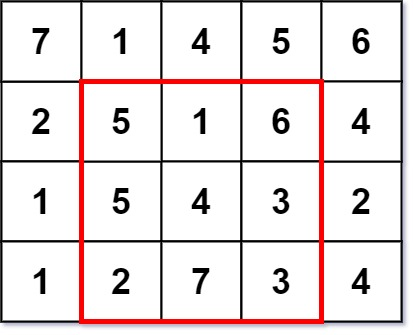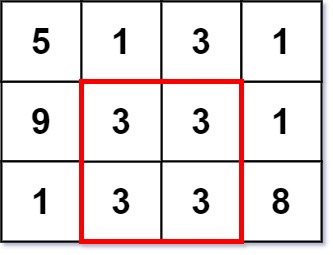# GeetCode Hub

A `k x k` magic square is a `k x k` grid filled with integers such that every row sum, every column sum, and both diagonal sums are all equal. The integers in the magic square do not have to be distinct. Every `1 x 1` grid is trivially a magic square.

Given an `m x n` integer `grid`, return the size (i.e., the side length `k`) of the largest magic square that can be found within this grid.

Example 1:```Input: grid = [[7,1,4,5,6],[2,5,1,6,4],[1,5,4,3,2],[1,2,7,3,4]]
Output: 3
Explanation: The largest magic square has a size of 3.
Every row sum, column sum, and diagonal sum of this magic square is equal to 12.
- Row sums: 5+1+6 = 5+4+3 = 2+7+3 = 12
- Column sums: 5+5+2 = 1+4+7 = 6+3+3 = 12
- Diagonal sums: 5+4+3 = 6+4+2 = 12
```

Example 2:```Input: grid = [[5,1,3,1],[9,3,3,1],[1,3,3,8]]
Output: 2
```

Constraints:

• `m == grid.length`
• `n == grid[i].length`
• `1 <= m, n <= 50`
• `1 <= grid[i][j] <= 106`

class Solution { public int largestMagicSquare(int[][] grid) { } }